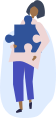Chemistry Homework Help

Get step-by-step solutions from expert tutors.

## How it works?Type, take a picture, or paste your question with all necessary details to ensure high-quality responses.### Get an answer in less than 24hrs

Once our tutors receive the question, they'll begin. You'll get an email once the solution is ready.### Get Notified

We will send you email and text notification to let you know when your question is answered.

### Browse Homework Solutions

127 solutions

Net Ionic Equations

#### Q. For problems 7-9, create balanced equations, state whether or not a chemical reaction occurs, and include the states of each reactant and product. If a precipitation reaction occurs, provide the ionic and net ionic equations. 9. Barium hydroxide solution is mixed with zinc (II) iodide solution to yield:

Solved • Feb 13, 2020

Net Ionic Equations

#### Q. For problems 7-9, create balanced equations, state whether or not a chemical reaction occurs, and include the states of each reactant and product. If a precipitation reaction occurs, provide the ionic and net ionic equations. 8. Ammonium sulfate solution is mixed with a barium hydroxide solution to yield:

Solved • Feb 13, 2020

Net Ionic Equations

#### Q. For problems 7-9, create balanced equations, state whether or not a chemical reaction occurs, and include the states of each reactant and product. If a precipitation reaction occurs, provide the ionic and net ionic equations. 7. A sodium chloride solution is mixed with a potassium hydroxide solution to yield:

Solved • Feb 13, 2020

Net Ionic Equations

#### Q. Identify from the following mixtures (problem 4-6) the ones that will produce a precipitate in water. Write balanced chemical reactions for those producing a precipitate (identify all species appropriately). Provide total ionic and net ionic equations: 6. Na2CO3 + CaI2:

Solved • Feb 13, 2020

Net Ionic Equations

#### Q. Identify from the following mixtures (problem 4-6) the ones that will produce a precipitate in water. Write balanced chemical reactions for those producing a precipitate (identify all species appropriately). Provide total ionic and net ionic equations: 5. LiOH + CaBr2:

Solved • Feb 13, 2020

Net Ionic Equations

#### Q. Identify from the following mixtures (problem 4-6) the ones that will produce a precipitate in water. Write balanced chemical reactions for those producing a precipitate (identify all species appropriately). Provide total ionic and net ionic equations: 4. AgNO3 + CaCl2:

Solved • Feb 13, 2020

Net Ionic Equations

#### Q. Given the following information: Acetic acid = CH3COOH Hypochlorous acid = HClO CH3COOH is a stronger acid than HClO (1) Write the net ionic equation for the reaction that occurs when equal volumes of 0.064 M acetic acid and sodium hypochlorite are mixed. It is not necessary to include states such as (aq) or (s). (2) Which is the strongest base, CH3COO- or ClO- (3) At equilibrium the __ will be favored.

Solved • Feb 16, 2020